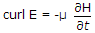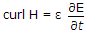# Electronics and Communication Engineering - Electromagnetic Field Theory

31.
A square corner reflector is used in which of the following?
Point-to-point communication
All of the above
Explanation:
No answer description is available. Let's discuss.

32.
Which of the following statement is incorrect?
curl E = -μ jωENone of the above
Explanation:
No answer description is available. Let's discuss.

33.
High frequency waves are
absorbed by the F2 layer
reflected by the D layer
affected by the solar cycle
none of the above
Explanation:
No answer description is available. Let's discuss.

34.
The direction of vectors gives the direction of propagation of electromagnetic waves
Vector (E x H)
Vector (F x H)
Vector E
Vector H